## LetsPlayMaths.Com

WELCOME TO THE WORLD OF MATHEMATICS

# Class 5 Multiples and Factors

Factors

Multiples

Divisibility Rules

Prime Numbers

Composite Numbers

Coprime Numbers

Highest Common Factors (HCF)

Least Common Factors (LCM)

Relation Between LCM & HCF

Multiples and Factors Test

Multiples and Factors Worksheet

## Factors

Let us consider a number 12 and find out it�s factors. Here, we must find out all the numbers by which 12 can be divisible completely.

1 X 12 = 12

2 X 6 = 12

3 X 4 = 12

So, factors of 12 are 1, 2, 3, 4, 6 and 12.

Let us consider another number, say, 24.

1 X 24 = 24

2 X 12 = 24

3 X 8 = 24

4 X 6 = 24

So, factors of 24 are 1, 2, 3, 4, 6, 8, 12 and 24.

## Multiples

3 X 4 = 12, we say 12 is multiple of 3 as well as 4.

4 X 6 = 24, here 24 is multiple of 4 as well as 6.

5 X 7 = 35, here 35 is multiple of 5 as well as 7.

Multiples of 2 are 2, 4, 6, 8, 10, 12, 14, 16, 18, ...

Multiples of 3 are 3, 6, 9, 12, 15, 18, 21, 24, 27, 30, ...

Multiples of 4 are 4, 8, 12, 16, 20, 24, 28, 32, 36, 40, ...

## Divisibility Rules

Let us recall the divisibility rules learnt in class IV.

a) A number which ends with 0, 2, 4, 6 or 8 is divisible 2.

b) A number is divisible by 3 when the sum of its digits is divisible by 3.

c) A number is divisible by 4 if the number formed by the last two digits on its extreme right is divisible by 4.

d) A number is divisible by 5 if its last digit is 0 or 5.

e) A number is divisible by 6, if it is divisible by both 2 and 3. We first check if it is divisible by 2 and then by 3.

f) A number is divisible by 8, if the number formed by its last three digits on its extreme right is divisible by 8.

g) A number is divisible by 9, if the sum of its digits is divisible by 9.

h) A number is divisible by 10, if the number's ones place digit is zero.

We have known above rules in class IV, let us learn divisibility rule for 7 and 11.

Divisibility Rule for 7

In order to find if a 2-digit number is divisible by 7, multiply the digit in the tens place by 3 and add the product to the digit in the ones place. If the result is divisible by 7, then the number is also divisible by 7.

Example 1. Is 84 is divisible by 7?

Solution. (8 X 3) + 4 = 24 + 4 = 28

We know, 28 is divisible by 7, so, 84 is also divisible by 7.

84 ÷ 7 = 12

To know if a three digit or larger number is divisible by 7, we take the last two digits of the number and add

it to the product of number formed by remaining digits and 2.

Example 2. Is 175 divisible by 7?

Solution. 75 + (1 X2) = 75 + 2 = 77

77 ÷ 7 = 11, 77 is divisible by 7.

So, 175 is also divisible by 7, 175 ÷ 7 = 25.

Example 3. Is 1092 is divisible by 7?

Solution. 92 + (10 X 2) = 92 + 20 = 112

Let�s check if 112 is divisible by 7

12 + (1 X 2) = 12 + 2 = 14

14 is divisible by 7, so are 112 and 1092.

Divisibility Rule for 11

A number is divisible by 11, if the difference between the sum of digits at the odd places in the number and the sum of digits at the even places in the number is either zero or divisible by 11.

Example 1. Find if 1771 is divisible by 11.

Solution. Sum of digits at the odd place = 1 + 7 = 8

Sum of digits at the even place = 1 + 7 = 8

Difference between sum of digits at the odd place and sum of digits at the even place is zero.

Thus, 1771 is divisible by 11.

Example 2. Find out if 61919 is divisible by 11.

Solution. Sum of digits at the odd place = 9 + 9 + 6 = 24

Sum of digits at the even place = 1 + 1 = 2

Difference of sums = 24 − 2 = 22

22 is divisible by 11, thus, 61919 is divisible by 11.

## Prime Numbers

A number which is divisible by 1 and itself are called Prime Numbers.

Example 1. Find out if 59 is prime number.

Solution.59 is not divisible by 2, 3, 4, 5, 6, 7, 8, 9, ... it is only divisible by 1 and itself.

Thus, 59 is a prime number.

## Composite Numbers

A number that is not a prime number is a composite number. A composite number has at least on factor other than one and itself.

Example 1. Is 56 a composite number?

Solution. 28 X 2 = 56 8 X 7 = 56

So, factors of 56 are 1, 2, 7, 8, 28 and 56. 56 is a composite number as it has factors other than 1 and 56.

## Coprime Numbers

Two numbers will be known as coprime numbers when they have only 1 as common factor.

Example 1. Are 16 and 45 coprime numbers?

Solution. Factors of 16 = 2 X 2 X 2 X 2 X 1

Factors of 45 = 1 X 3 X 3 X 5

1 is the common factors of 16 and 45. So, both 16 and 45 are coprime numbers.

## Highest Common Factors (HCF)

In class IV, we have learnt about HCF. It is the greatest number which divides the given numbers fully without leaving any remainder. We can find out HCF by using following methods.

1. Listing Method

2. Prime Factorizing Method

3. Division Method

Listing Method

Example 1. Find HCF of 156 and 248.

Solution. Factors of 156 are = 1, 2, 3, 4, 12, 13, 39, 52, 78 and 156

Factors of 248 are = 1, 2, 4, 8, 31, 62, 124 and 248

Highest common factor of 156 and 248 is 4.

Example 2. Find HCF of 64, 128 and 256.

Solution. Factors of 64 are = 1, 2, 4, 8, 16, 32 and 64

Factors of 128 = 1, 2, 4, 8, 16, 32, 64 and 128

Factors of 256 = 1, 2, 4, 8, 16, 32, 64, 128 and 256

Highest common factor of 64, 128 and 256 is 64.

Prime Factorizing Method

Example 1. Find HCF of 32 and 48.

Solution.Factorize both the numbers until they have common factors

So, HCF of 32 and 48 = 2 X 2 X 2 X 2 = 16

Example 2. Find HCF of 81, 108 and 135.

Solution.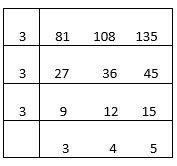Factorize both the numbers until they have common factors.

So, HCF of 81, 108 and 135 = 3 X 3 X 3 = 27

Division Method
We have learnt Division Method of Finding HCF in class IV. This method is more useful when the numbers are large. To find out the HCF of two numbers, we take the following steps given below.

a) First divide the larger number by smaller number.

b) Next, divide the previous divisor by the remainder in step A.

c) We then divide the new divisor of step B by the new remainder by the new remainder in step B.

d) Continue the process like step B till we get zero as remainder.

e) We write the last divisor as HCF of given numbers.

Example 1. Find the HCF of 882 and 648.

Solution.18 is the HCF of 648 and 882.

Example 2. Find the HCF of 255, 289, 340.

Solution.HCF of 289 and 340 is 17.

Let�s find out HCF of 17 and 255.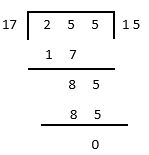So, the HCF of 255, 289 and 340 is 17.

## Least Common Factors (LCM)

The least common multiple of two or more numbers is the lowest numbers which is exactly divisible by those numbers. We can find out LCM in two methods.

1. Listing Method

2. Division Method

Listing Method

In this method, we must list out all the multiples of given numbers, then find out the lowest common multiples.

Example 1. Find out LCM of 4 and 5.

Solution.

Multiples of 4 are 4, 8, 12, 14, 20, 24, 28, 32, 36, 40, ...

Multiples of 5 are 5, 10, 15, 20, 25, 30, 35, 40, 45, 50, ...

From the above list we can see 20 and 40 are common multiples of 4 and 5, and 20 is the Lowest Common Multiples.

So, 20 is the LCM of 4 and 5.

Example 2. Find out LCM of 6 and 8.

Solution.

Multiples of 6 are 6, 12, 18, 24, 30, 42, 48, 54, 60, 66, 72, ...

Multiples of 8 are 8, 16, 24, 32, 40, 48, 56, 64, 72, 80, 88, ...

24, 48 and 72 are common multiples of 6 and 8.

24 is the Lowest Common Multiple of 6 and 8.

Division Method

In this method, we take the numbers and divide them with the smallest possible prime factor. If any number gets divided fully then we divide it, else we keep it as it is. In the next step, we divide it by the next possible prime factor. We keep on doing it unless we get all the quotients become 1. In the end, we multiply all the prime factors to get the LCM.

Example 1. Find the LCM of 8, 16, 24, and 32.

Solution.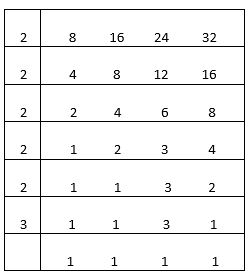So, the LCM of 8, 16, 24 and 32 = 2 X 2 X 2 X 2 X 2 X 3 = 96

Example 2. Find the LCM of 120, 140 and 160.

Solution.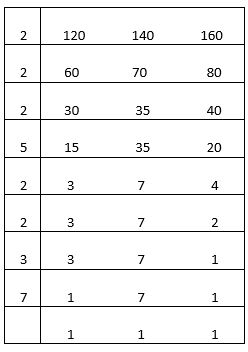So, the LCM of 120, 140 and 160 = 2 X 2 X 2 X 5 X 2 X 2 X 3 X 7 = 3360

## Relation Between LCM & HCF

Product of two numbers = HCF X LCM

Let's see some example on the above-mentioned relation.

Example 1. Find out the product of 8 and 16 by using LCM and HCF.

Solution.

LCM of 8 and 16.LCM of 8 and 16 = 2 X 2 X 2 X 3 = 24

HCF of 8 and 16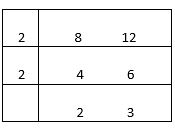HCF of 8 and 16 = 2 X 2

Product of 12 and 8 = LCM X HCF = 24 X 4 = 96

Product of 12 and 8 = 12 X 8 = 96

Example 2. Find out the product of 72 and 80 by using LCM and HCF.

Solution.

LCM of 72 and 80.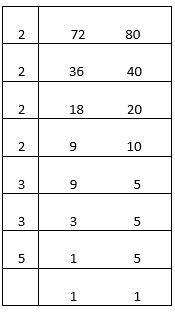LCM of 72 and 80 = 2 X 2 X 2 X 2 X 3 X 3 X 5 = 720

HCF of 72 and 80.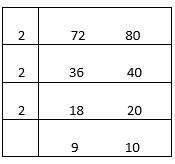HCF of 72 and 80 = 2 X 2 X 2 = 8

Product of 72 and 80 = LCM X HCF = 720 X 8 = 5760

Product of 72 and 80 = 72 X 80 = 5760

## Multiples and Factors Test

Multiples & Factors Test - 1

Multiples & Factors Test - 2

## Class-5 Multiples and Factors Worksheet

Multiples & Factors Worksheet - 1

Multiples & Factors Worksheet - 2

Multiples & Factors Worksheet - 3

Multiples & Factors Worksheet - 4

Multiples & Factors Worksheet - 5

Multiples & Factors Worksheet - 6